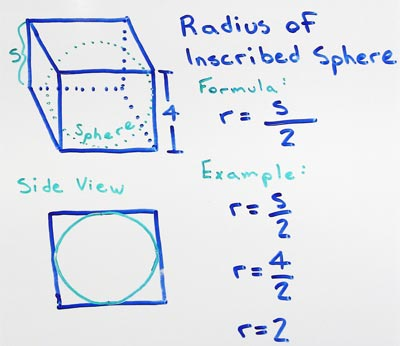# Volume of Cube

## - Cube Inscribed Sphere Radius

A cube with a side length (S) of 4 has an inscribed sphere with a radius (R) of 2.

for cubes with a side length S find the radius R of the inscribed sphere

• R = S/2

substitute the side length S with the measured value, in this example lets use a side length of 4

• R = 4/2

divide the side length in half to find the radius of an inscribed sphere

• R = 2Given a cube with a side length S the radius (R) of an inscribed sphere can be found by dividing the cube side length in half.

### Formulas

What is the formula for the volume of a Cube?

V = S3

What is the formula for the volume of a Rectangular Prism?

V = L x W x H

### Other Formulae

for cubes with side length S

Cube Surface Area

6S2
Cube Face Diagonal
(√2)S
Cube Space Diagonal
(√3)S
S/2
All formulae...